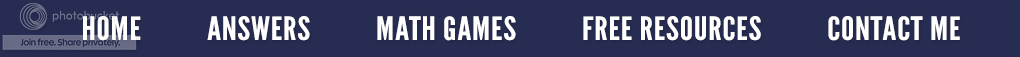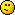### Math or Maths?

I keep seeing the word "maths" on Pinterest.To me it is confusing and puzzling in addition to being a misspelling or misuse of the word "math".  Where in the world did this word come from, who created it, and why?

Math can be used in the singular form ("math") or in the collective form ("math") without distinction. Math is a field of study which is divided into specific multiple disciplines such as algebra, geometry, etc. which then are again divided into mathematical subgroups.

Likewise, "physics" is single word for a particular field of study, even though it ends in "s". You don't study "a physic"; you study "physics". Just like physics, mathematics is considered singular, but maths? Isn't that like saying deers for deer or sheeps for sheep?

Well, here is the mathematical truth.  Maths is the term commonly used in England, Australia, New Zealand, etc.  It is a shortened form of mathematics.  They pluralize the word, and refer to studying "maths" because mathematics has an "s" on the end.  So the answer to this "maths" question is that it depends on where you live as to what word you use.  Therefore be aware of the geographical differences so you can use the correct form of the word in your writing or speaking.  And when you see the word "maths", don't jump to the conclusion it is a misspelling or a misuse.  Recognize that the writer most likely lives outside of the United States.

Interested in "Math" stuff?  There are 51 resources in my store just for math.  Just go to the category of Math Stuff.